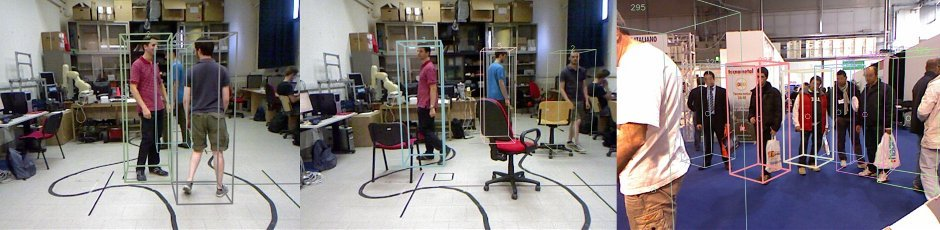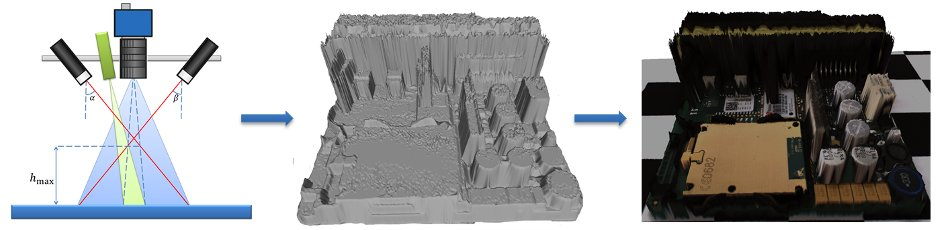•••••﻿

1. Levente Hunyadi. Poster at a conference. Poster at Automation and Applied Computer Science Workshop, Budapest, Hungary, 2016. A short note for the poster. BibTeX

```@poster{key:poster,
title = "Poster at a conference",
year = 2016,
event = "Automation and Applied Computer Science Workshop",
location = "Budapest, Hungary",
note = "A short note for the poster",
abstract = "A short summary of the poster paper publication."
}
```
2. Levente Hunyadi. PhDThesis: A PhD thesis. Final version, Budapest University of Technology and Economics, Budapest, January 2014. A short note. BibTeX

```@phdthesis{key:phdthesis,
title = "PhDThesis: A PhD thesis",
school = "Budapest University of Technology and Economics",
year = 2014,
type = "Final version",
month = "January",
note = "A short note",
abstract = "A short summary of the publication."
}
```
3. Levente Hunyadi. Unpublished: A document having an author and title, but not formally published. December 2009, A short note. URL PDF BibTeX

```@unpublished{key:unpublished,
title = "Unpublished: A document having an author and title, but not formally published",
note = "A short note",
month = "December",
year = 2009,
}
```
4. Levente Hunyadi. TechReport: A report published by a school or other institution, usually numbered within a series. Final version 2, Department of Automation and Applied Informatics, December 2009. A short note. BibTeX

```@techreport{key:techreport,
title = "TechReport: A report published by a school or other institution, usually numbered within a series",
institution = "Department of Automation and Applied Informatics",
year = 2009,
type = "Final version",
number = 2,
month = "December",
note = "A short note",
abstract = "A short summary of the publication."
}
```
5. István Vajk (ed.). Proceedings: The proceedings of a conference 1(2). Budapest University of Technology and Economics, BME Press, Budapest, December 2009. A short note. BibTeX

```@proceedings{key:proceedings,
title = "Proceedings: The proceedings of a conference",
year = 2009,
editor = "Istv\'an Vajk",
volume = 1,
number = 2,
series = 3,
month = "December",
organization = "Budapest University of Technology and Economics",
publisher = "BME Press",
note = "A short note",
abstract = "A short summary of the publication."
}
```
6. Levente Hunyadi. Misc: Use this type when nothing else fits. Published electronically, December 2009. A short note. BibTeX

```@misc{key:misc,
title = "Misc: Use this type when nothing else fits",
howpublished = "Published electronically",
month = "December",
year = 2009,
note = "A short note",
abstract = "A short summary of the publication."
}
```
7. Levente Hunyadi. Manual: Technical documentation. Budapest University of Technology and Economics, Budapest, second edition, December 2009. A short note. BibTeX

```@manual{key:manual,
title = "Manual: Technical documentation",
organization = "Budapest University of Technology and Economics",
edition = "Second",
month = "December",
year = 2009,
note = "A short note",
abstract = "A short summary of the publication."
}
```
8. Levente Hunyadi. InCollection: A part of a book having its own title. In István Vajk (ed.). A book with an explicit publisher. Third series, volume 1, number 2, BME Press, December 2009, pages 99–999. A short note. BibTeX

```@incollection{key:incollection,
title = "InCollection: A part of a book having its own title",
booktitle = "A book with an explicit publisher",
publisher = "BME Press",
year = 2009,
editor = "Istv\'an Vajk",
volume = 1,
number = 2,
series = 3,
type = "Final version",
chapter = 4,
pages = "99--999",
edition = "Second",
month = "December",
note = "A short note",
abstract = "A short summary of the publication."
}
```
9. István Vajk (ed.). InBook: A part of a book, which may be a chapter and/or a range of pages. Final version, third series, volume 1, number 2, chapter 4, pages 99–999, BME Press, December 2009. A short note. BibTeX

```@inbook{key:inbook,
editor = "Istv\'an Vajk",
title = "InBook: A part of a book, which may be a chapter and/or a range of pages",
chapter = 4,
pages = "99--999",
publisher = "BME Press",
year = 2009,
volume = 1,
number = 2,
series = 3,
type = "Final version",
edition = "Second",
month = "December",
note = "A short note",
abstract = "A short summary of the publication."
}
```
10. István Vajk (ed.). Book: A book with an explicit publisher. Second edition, third series, volume 1, number 2, BME Press, December 2009. A short note. BibTeX

```@book{key:book,
editor = "Istv\'an Vajk",
title = "Book: A book with an explicit publisher",
publisher = "BME Press",
year = 2009,
volume = 1,
number = 2,
series = 3,
edition = "Second",
month = "December",
note = "A short note",
abstract = "A short summary of the publication."
}
```
11. Levente Hunyadi. Booklet: A work that is printed and bound, but without a named publisher or sponsoring institution. Available electronically, Budapest, October 2009. A short note. BibTeX

```@booklet{key:booklet,
title = "Booklet: A work that is printed and bound, but without a named publisher or sponsoring institution",
howpublished = "Available electronically",
month = "October",
year = 2009,
note = "A short note",
abstract = "A short summary of the publication."
}
```
12. Levente Hunyadi. Article: An article from a journal or magazine. The BibTeX Journal 1(2):99–999, May 2009. A short note. BibTeX

```@article{key:article,
title = "Article: An article from a journal or magazine",
journal = "The BibTeX Journal",
year = 2009,
volume = 1,
number = 2,
pages = "99--999",
month = "May",
note = "A short note",
abstract = "A short summary of the publication."
}
```
13. Levente Hunyadi. InProceedings: An article in a conference proceedings. In István Vajk (ed.). A book with an explicit publisher 1(2). January 2009, 99–999. A short note. BibTeX

```@inproceedings{key:inproceedings,
title = "InProceedings: An article in a conference proceedings",
booktitle = "A book with an explicit publisher",
year = 2009,
editor = "Istv\'an Vajk",
volume = 1,
number = 2,
series = 3,
pages = "99--999",
month = "January",
organization = "Budapest University of Technology and Economics",
publisher = "BME Press",
note = "A short note",
abstract = "A short summary of the publication."
}
```
14. Levente Hunyadi. MastersThesis: A Master's thesis. Final version, Budapest University of Technology and Economics, Budapest, June 2007. A short note. BibTeX

```@mastersthesis{key:mastersthesis,
title = "MastersThesis: A Master's thesis",
school = "Budapest University of Technology and Economics",
year = 2007,
type = "Final version",
month = "June",
note = "A short note",
abstract = "A short summary of the publication."
}
```
15. "The @ symbol". "Quotation marks". BibTeX

```@unpublished{key:special,
title = "{"}The @ symbol{"}",
note = {"Quotation marks"}
}
```
16. List of symbols. \$ % & # ¶ § _ © † ‡ … £ å Å Æ æ Ð ð Đ đ Ł ł Ŋ ŋ Ø ø Œ œ ß SS Þ þ. BibTeX

```@unpublished{key:symbols,
title = "List of symbols",
note = "\\$ \% \& \# \P \S \_ \copyright \dag \ddag \dots \pounds \{ \} \AA \aa \AE \ae \DH \dh \DJ \dj \L \l \NG \ng \O \o \OE \oe \ss \SS \TH \th"
}
```
17. Árvíztűrő tükörfúrógép. Á á Á á Ǽ ǽ Ǽ ǽ É é É é Í í Í í Ó ó Ó ó Ú ú Ú ú Ć ć Ć ć Ĺ ĺ Ĺ ĺ Ń ń Ń ń Ŕ ŕ Ŕ ŕ Ś ś Ś ś Ẃ ẃ Ẃ ẃ Ý ý Ý ý Ź ź Ź ź À à À à È è È è Ì ì Ì ì Ò ò Ò ò Ù ù Ù ù Ẃ ẃ Ẃ ẃ Ý ý Ý ý Â â Â â Ê ê Ê ê Î î Î î Ô ô Ô ô Û û Û û Ĉ ĉ Ĉ ĉ Ĝ ĝ Ĝ ĝ Ĥ ĥ Ĥ ĥ Ĵ ĵ Ĵ ĵ Ŝ ŝ Ŝ ŝ Ŵ ŵ Ŵ ŵ Ŷ ŷ Ŷ ŷ Ä ä Ä ä Ë ë Ë ë Ï ï Ï ï Ö ö Ö ö Ü ü Ü ü Ẅ ẅ Ẅ ẅ Ÿ ÿ Ÿ ÿ Ǎ ǎ Ě ě Ǐ ǐ Ǒ ǒ Ǔ ǔ Č č Ď ď Ľ ľ Ň ň Ř ř Š š Ť ť Ž ž Ã ã Ã ã Ã ã Ã ã Ĩ ĩ Ĩ ĩ Õ õ Õ õ Ũ ũ Ũ ũ Ñ ñ Ñ ñ Ỹ ỹ Ỹ ỹ Ç ç Ģ ģ Ķ ķ Ļ ļ Ņ ņ Ŗ ŗ Ş ş Ţ ţ Ő ő Ű ű. BibTeX

```@unpublished{key:accents,
title = {{\'A}rv\'izt{\H{u}}r{\H{o}} t\"uk\"orf\'ur\'og\'ep},
note = {\'A \'a \'{A} \'{a} \'\AE \'\ae \'{\AE} \'{\ae} \'E \'e \'{E} \'{e} \'I \'i \'{I} \'{i} \'O \'o \'{O} \'{o} \'U \'u \'{U} \'{u} \'C \'c \'{C} \'{c} \'L \'l \'{L} \'{l} \'N \'n \'{N} \'{n} \'R \'r \'{R} \'{r} \'S \'s \'{S} \'{s} \'W \'w \'{W} \'{w} \'Y \'y \'{Y} \'{y} \'Z \'z \'{Z} \'{z} \`A \`a \`{A} \`{a} \`E \`e \`{E} \`{e} \`I \`i \`{I} \`{i} \`O \`o \`{O} \`{o} \`U \`u \`{U} \`{u} \'W \'w \'{W} \'{w} \'Y \'y \'{Y} \'{y} \^A \^a \^{A} \^{a} \^E \^e \^{E} \^{e} \^I \^i \^{I} \^{i} \^O \^o \^{O} \^{o} \^U \^u \^{U} \^{u} \^C \^c \^{C} \^{c} \^G \^g \^{G} \^{g} \^H \^h \^{H} \^{h} \^J \^j \^{J} \^{j} \^S \^s \^{S} \^{s} \^W \^w \^{W} \^{w} \^Y \^y \^{Y} \^{y} \"A \"a \"{A} \"{a} \"E \"e \"{E} \"{e} \"I \"i \"{I} \"{i} \"O \"o \"{O} \"{o} \"U \"u \"{U} \"{u} \"W \"w \"{W} \"{w} \"Y \"y \"{Y} \"{y} \v{A} \v{a} \v{E} \v{e} \v{I} \v{i} \v{O} \v{o} \v{U} \v{u} \v{C} \v{c} \v{D} \v{d} \v{L} \v{l} \v{N} \v{n} \v{R} \v{r} \v{S} \v{s} \v{T} \v{t} \v{Z} \v{z} \~A \~a \~{A} \~{a} \~A \~a \~{A} \~{a} \~I \~i \~{I} \~{i} \~O \~o \~{O} \~{o} \~U \~u \~{U} \~{u} \~N \~n \~{N} \~{n} \~Y \~y \~{Y} \~{y} \c{C} \c{c} \c{G} \c{g} \c{K} \c{k} \c{L} \c{l} \c{N} \c{n} \c{R} \c{r} \c{S} \c{s} \c{T} \c{t} \H{O} \H{o} \H{U} \H{u}}
}
```